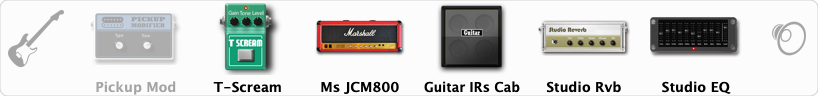# JCM 800 crunch

Discussion in 'ToneLib-GFX presets' started by Jozef Stiavnicky, Jun 28, 2019.

1. ### Jozef StiavnickyNew Member

JCM 800 crunch
Preset name: JCM 800 crunch

Nice old MS

Effects chain:Effect: "Pickup Mod" (Dynamics / Filter), active - "no"
"Mode" = Humbacker->Single
"Tone" = 72

Effect: "T-Scream" (Overdrive / Distortion), active - "yes"
"Drive" = 58
"Tone" = 30
"Level" = 50

Effect: "Ms JCM800" (Amp simulators), active - "yes"
"Gain" = 58
"Bass" = 90
"Middle" = 58
"Treble" = 58
"Presence" = 30
"Master" = 58
"Output" = 50
"Level (dB)" = 2

Effect: "Guitar IRs Cab" (Cabinets), active - "yes"
"Model" = Marshall 1960A (4x12")
"Mic Position" = Middle
"Mic Distance" = Near
"Low Cut (Hz)" = 60
"Hi Cut (kHz)" = 20.0
"Mix" = 100
"Level (dB)" = 0

Effect: "Studio Rvb" (Reverb), active - "yes"
"Time" = 7.4
"PreDelay" = 32
"LoDamp" = 0
"HiDamp" = 12
"Mix" = 29

Effect: "Studio EQ" (Dynamics / Filter), active - "yes"
"31 Hz" = -3
"62 Hz" = 0
"125 Hz" = 3
"250 Hz" = 4
"500 Hz" = 10
"1 kHz" = 8
"2 kHz" = 6
"4 kHz" = 3
"8 kHz" = -2
"16 kHz" = -15
"above 16 kHz" = 0
"Level (dB)" = -5

Note: You will need to download and install the ToneLib-GFX software to use the preset.

#### Attached Files:

• ###### JCM_800_crunch.tlgfx
File size:
597 bytes
Views:
4,813
Last edited: Jul 12, 2019